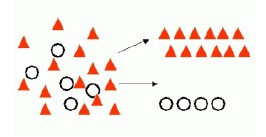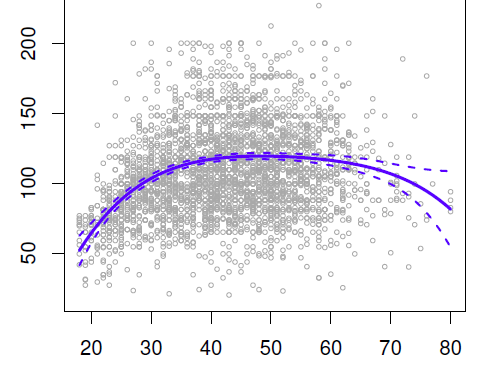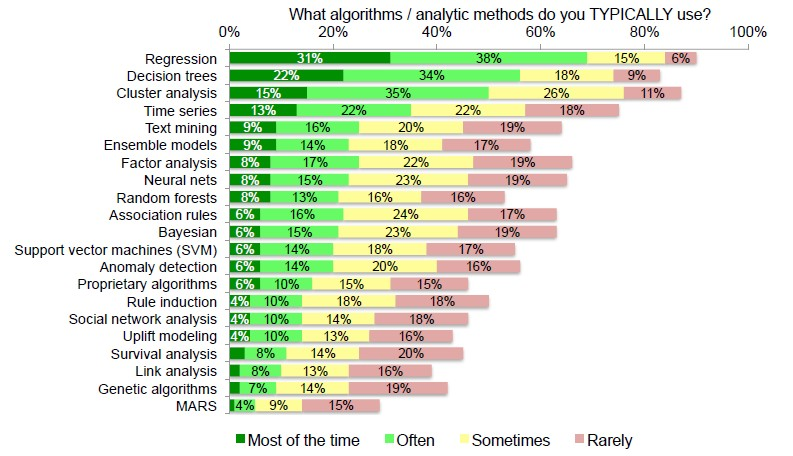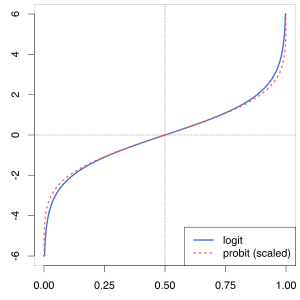# Statistics - Regression

Regression is a statistical analysis used:

• to predict scores on an numeric outcome variable,
• based on scores of:
• one predictor variable: simple regression
• or multiple predictor variables: multiple regression

Regression analysis is a statistical process (Supervised function) for:

“Regression” problems are principally aimed to resolve problem with a continuous value (numeric) outcome but can also be applied to nominal outcome

Regression analysis helps one understand how the typical value of a (outcome|dependent) variable changes when any one of the (predictor|independent) variables is varied, while the other (predictor|independent) variables are held fixed. (ie which among the independent variables are related to the dependent variable)

The term “regression” was coined by Francis Galton in the nineteenth century to describe a biological phenomenon. The phenomenon was that the heights of descendants of tall ancestors tend to regress down towards a normal average (a phenomenon also known as regression toward the mean). For Galton, regression had only this biological meaning, but his work was later extended by Udny Yule and Karl Pearson to a more general statistical context.

“Regression” comes historically from the idea of regression towards the mean, which is a concept which was discussed in the early 1900s. But we have to live with this term because it's become time honored and you can change this term by model.

## Example

• Given demographic and purchasing data about a set of customers, predict customers' age
• Customer lifetime value, house value, process yield rates

## Algorithm

Many (techniques|methods) for carrying out regression analysis have been developed.

Technique Parametric Description
Nearest Neighbors No
Linear regression Yes
Data Mining - (Global) Polynomial Regression (Degree) Yes
Statistics - Standard Least Squares Fit (Gaussian linear model)
ordinary least squares regression Yes The earliest form of regression which was published by Legendre in 1805 and by Gauss in 1809.
Multiple Regression (GLM)
Support Vector Machine (SVM)
Logistic regression
LeastMedSq LeastMedSq gives an accurate regression line even when there are outliers. However, it is computationally very expensive. In practical situations it is common to delete outliers manually and then use LinearRegression.

## Model

A model predicts a specific target value for each case from among (possibly) infinitely many values.

The regression model is used to model or predict future behaviour and involves the following variables:

$$Y = f(X) + \epsilon$$

• or from Wikipedia

$$\begin{array}{ccc} Y & \approx & f ( {X}, \beta ) \\ E(Y | X) & = & f ( {X}, \beta ) \end{array}$$ The approximation is usually formalized as E(Y | X)

The true function above generates always errors, When there is no error, there is overfitting.

A model is:

• simple: the model is the the regression equation.
• or complex: the model is a set of regression equations

Example of Simple Linear Regression Model: $Model = \hat{Y} = B_0 + B_1.{X_1}$

### How to improve the model ?

The goal is to produce better models so we can generate more accurate predictions

We can improve a model by:

• Adding more predictor variables (but it will add overfitting and variance.)
• Developing better predictor variables with more reliable measures or more valid measures of the construct.
• Selecting feature. When we want to predict better, we'll shrink, or regularize, or select features in order to improve the prediction.

## Inferential statistics

When we're doing regression, we're more engaging in inferential statistics and we're going to look at this statistics:

• p value (in order to make probabilities judgement)

in order to know if the results from this sample is going to generalize to other samples.

We want to know if it's possible to make an inference from this sample data to a more general population.

The lm function

## Documentation / Reference

Discover More(Machine|Statistical) Learning - (Target|Learned|Outcome|Dependent|Response) (Attribute|Variable) (Y|DV)

An (outcome|dependent) variable is ameasure that we want to predict. : the original score collected : the predicted score (or estimator) from the equation. The hat means “estimated” from the...Analytic (Data Analyst)

A Data Analyst sees what data he has. A Data Scientist imagines what data he is lacking. Information analysis provides insight into : the past the present and the future of the business. See...Data Mining - (Classifier|Classification Function)

A classifier is a Supervised function (machine learning tool) where the learned (target) attribute is categorical (“nominal”) in order to classify. It is used after the learning process to classify...Data Mining - (Class|Category|Label) Target

A class is the category for a classifier which is given by the target. The number of class to be predicted define the classification problem. A class is also known as a label. Labeled...Data Mining - (Discriminative|conditional) models

Discriminative models, also called conditional models, are a class of models used in machine learning for modeling the dependence of an unobserved variable y on an observed variable x. Discriminative...Data Mining - (Function|Model)

The model is the function, equation, algorithm that predicts an outcome value from one of several predictors. During the training process, the models are build. A model uses a logic and one of several...Data Mining - (Global) Polynomial Regression (Degree)

polynomials regression Although polynomials are easy to think of, splines are much better behaved and more local. With polynomial regression, you create new variables that are just transformations...Data Mining - (Prediction|Guess)

Something predictable is showing a pattern and is therefore not truly random. entropytrue randomness Many forms of data mining model are predictive. For example, a model might predict income based on...Data Mining - Algorithms

An is a mathematical procedure for solving a specific kind of problem. For some data mining functions, you can choose among several algorithms. Algorithm Function Type Description Decision...Data Mining - Probit Regression (probability on binary problem)

Probit_modelprobit model (probability + unit) is a type of regression where the dependent variable can only take two values. As the Probit function is really similar to the logit function, the probit...